• Logic Unit, ALU)是中央处理器(CPU)执行单元，是所有中央处理器核心组成部分，由"And Gate" 和"Or Gate"构成算术逻辑单元，主要功能是进行二进制算术运算，如加减乘(不包括整数除法)。基本上，所有现代...
算术逻辑单元 (Arithmetic
Logic Unit, ALU)是中央处理器(CPU)的执行单元，是所有中央处理器的核心组成部分，由"And Gate" 和"Or Gate"构成的算术逻辑单元，主要功能是进行二进制的算术运算，如加减乘(不包括整数除法)。基本上，在所有现代CPU体系结构中，二进制都以二补数的形式来表示。

定义
计算机中执行各种算术和逻辑运算操作的部件。运算器的基本操作包括加、减、乘、除四则运算，与、或、非、异或等逻辑操作，以及移位、比较和传送等操作，亦称算术逻辑部件（ALU）。计算机运行时，运算器的操作和操作种类由控制器决定。运算器处理的数据来自存储器；处理后的结果数据通常送回存储器，或暂时寄存在运算器中。

A

数据运算器的处理对象是数据，所以数据长度和计算机数据表示方法，对运算器的性能影响极大。70年代微处理器常以1个、4个、8个、16个二进制位作为处理数据的基本单位。大多数通用计算机则以16、32、64位作为运算器处理数据的长度。能对一个数据的所有位同时进行处理的运算器称为并行运算器。如果一次只处理一位，则称为串行运算器。有的运算器一次可处理几位
（通常为6或8位），一个完整的数据分成若干段进行计算，称为串 并行运算器。运算器往往只处理一种长度的数据。有的也能处理几种不同长度的数据，如半字长运算、双倍字长运算、四倍字长运算等。有的数据长度可以在运算过程中指定，称为变字长运算。

按照数据的不同表示方法，可以有二进制运算器、十进制运算器、十六进制运算器、定点整数运算器、定点小数运算器、浮点数运算器等。按照数据的性质，有地址运算器和字符运算器等。

操作运算器能执行多少种操作和操作速度，标志着运算器能力的强弱，甚至标志着计算机本身的能力。运算器最基本的操作是加法。一个数与零相加，等于简单地传送这个数。将一个数的代码求补，与另一个数相加，相当于从后一个数中减去前一个数。将两个数相减可以比较它们的大小。

左右移位是运算器的基本操作。在有符号的数中，符号不动而只移数据位，称为算术移位。若数据连同符号的所有位一齐移动，称为逻辑移位。若将数据的最高位与最低位链接进行逻辑移位，称为循环移位。

运算器的逻辑操作可将两个数据按位进行与、或、异或，以及将一个数据的各位求非。有的运算器还能进行二值代码的16种逻辑操作。

寄存器连线面

乘、除法操作较为复杂。很多计算机的运算器能直接完成这些操作。乘法操作是以加法操作为基础的，由乘数的一位或几位译码控制逐次产生部分积，部分积相加得乘积。除法则又常以乘法为基础，即选定若干因子乘以除数，使它近似为1，这些因子乘被除数则得商。没有执行乘法、除法硬件的计算机可用程序实现乘、除，但速度慢得多。有的运算器还能执行在一批数中寻求最大数，对一批数据连续执行同一种操作，求平方根等复杂操作。

编辑本段运算方法
实现运算器的操作，特别是四则运算，必须选择合理的运算方法。它直接影响运算器的性能，也关系到运算器的结构和成本。另外，在进行数值计算时，结果的有效数位可能较长，必须截取一定的有效数位，由此而产生最低有效数位的舍入问题。选用的舍入规则也影响到计算结果的精确度。

编辑本段结构
运算器包括寄存器、执行部件和控制电路3个部分。

在典型的运算器中有3个寄存器：接收并保存一个操作数的接收寄存器；保存另一个操作数和运算结果的累加寄存器；在进行乘、除运算时保存乘数或商数的乘商寄存器。执行部件包括一个加法器和各种类型的输入输出门电路。控制电路按照一定的时间顺序发出不同的控制信号，使数据经过相应的门电路进入寄存器或加法器，完成规定的操作。

为了减少对存储器的访问，很多计算机的运算器设有较多的寄存器，存放中间计算结果，以便在后面的运算中直接用作操作数。

为了提高运算速度，某些大型计算机有多个运算器。它们可以是不同类型的运算器，如定点加法器、浮点加法器、乘法器等，也可以是相同类型的运算器。

编辑本段运算器
由算术逻辑单元(ALU)、累加寄存器、数据缓冲寄存器和状态条件寄存器组成，它是数据加工处理部件。相对控制器而言，运算器接受控制器的命令而进行动作
，即运算器所进行的全部操作都是由控制器发出的控制信号来指挥的所以它是执行部件。

编辑本段主要功能
执行所有的算术运算；

执行所有的逻辑运算，并进行逻辑测试，如零值测试或两个值的比较。

编辑本段下面举例8位ALU的两种设计方案
使用原理图方法设计：

使用VHDL方法设计：

8-Bit ALU in VHDL

This arithmetic logic unit accepts 8-bit inputs, but it can easily be modded to higher bits. It supports the addition, subtraction, set if less than, AND, and OR operations. The
operation to perform is determined by the 3-bit address bus.

library ieee;

use ieee.std_logic_1164.all;

use ieee.std_logic_unsigned.all;

use ieee.std_logic_arith.all;

ENTITY alu8bit IS

port(a, b : in std_logic_vector(7 downto 0); -- a and b are busses

op : in std_logic_vector(2 downto 0);

zero : out std_logic;

f : out std_logic_vector(7 downto 0));

END alu8bit;

architecture behavioral of alu8bit is

begin

process(op)

variable temp: std_logic_vector(7 downto 0);

begin

case op is

when "000" =>

temp := a and b;

when "100" =>

temp := a and b;

when "001" =>

temp := a or b;

when "101" =>

temp := a or b;

when "010" =>

temp := a + b;

when "110" =>

temp := a - b;

when "111" =>

if a < b then

temp := "11111111";

else

temp := "00000000";

end if;

when others =>

temp := a - b;

end case;

if temp="00000000" then

zero <= '1';

else

zero <= '0';

end if;

f <= temp;

end process;

end behavioral;

以此我们可以推出4位、32位等等的ALU设计方法。

展开全文vector 存储 library 测试 less
• 算术逻辑运算单元（ALU基本功能为加、减、乘、除四则运算，与、或、非、异或等逻辑操作，以及移位、求补等操作。计算机运行时，运算器操作和操作种类由控制器决定。运算器处理数据来自存储器；处理后结果...
• Logical Unit）**是中央处理器(CPU)执行单元，是所有中央处理器核心组成部分，由"And Gate"（与门） 和"Or Gate"（或门）构成算术逻辑单元，主要功能是进行二位元算术运算，如加减乘(不包括整数除法)。...
ALU设计ALU简介ALU实例运行结果
ALU简介
算术逻辑单元（Arithmetic&Logical Unit）是能实现多组算术运算和逻辑运算的组合逻辑电路，简称ALU。它是中央处理器(CPU)的执行单元，是所有中央处理器的核心组成部分，由门电路构成的算术逻辑单元，主要功能是进行二位元的算术运算，如加减乘(不包括整数除法)。基本上，在所有现代CPU体系结构中，二进制都以补码的形式来表示。
大部分ALU都可以完成以下运算∶
整数算术运算（加、减，有时还包括乘和除，不过成本较高）
位逻辑运算（与、或、非、异或）
移位运算（左移、右移）
如果在ALU中集成复杂运算，可以在内部采用流水线的方式实现。
ALU实例
以下展示一个简单的ALU模块的实例。
alu.v
timescale 1ns / 1ps

// Company:
// Engineer:
//
// Create Date: 2020/12/07
// Author Name: Sniper
// Module Name: alu
// Project Name:
// Target Devices:
// Tool Versions:
// Description:
//
// Dependencies:
//
// Revision:
// Revision 0.01 - File Created
//

module alu
#(
parameter DATA_WIDTH = 32
)
(
input [DATA_WIDTH-1:0] a,		//operand of ALU
input [DATA_WIDTH-1:0] b,		//operand of ALU
input cin,              		//carry in at the LSB
input [3:0] operate,			//operate select
output reg [DATA_WIDTH-1:0] r,	//result of ALU
output reg cout					//carry produced by ALU operation
);

localparam SUB = 4'b0001;//r=a-b
localparam AND = 4'b0010;//r=a&b
localparam OR  = 4'b0011;//r=a|b
localparam NOT = 4'b0100;//r=~a
localparam XOR = 4'b0101;//r=a^b
localparam COMPRAE = 4'b0110;//r=a>b?1:0
localparam SHIFT_L = 4'b0111;//r=a<<b
localparam SHIFT_R = 4'b1000;//r=a>>b

//function for calculation
function [DATA_WIDTH:0] calculate;
input [DATA_WIDTH-1:0] a;
input [DATA_WIDTH-1:0] b;
input cin;
input [3:0] oper;

begin
case(oper)
SUB: calculate = {1'b0, a-b};
AND: calculate = {1'b0, a&b};
OR : calculate = {1'b0, a|b};
NOT: calculate = {1'b0, ~a};
XOR: calculate = {1'b0, a^b};
COMPRAE: calculate = {1'b0, a>b?1:0};
SHIFT_L: calculate = {1'b0, a<<b};
SHIFT_R: calculate = {1'b0, a>>b};

default: calculate = 0;
endcase
end
endfunction

//calculate
always@(*)
begin
{cout, r[DATA_WIDTH-1:0]} = calculate(a, b, cin, operate);
end

endmodule

tb_alu.v
timescale 1ns / 1ps

// Company:
// Engineer:
//
// Create Date: 2020/12/07
// Author Name: Sniper
// Module Name: tb_alu
// Project Name:
// Target Devices:
// Tool Versions:
// Description:
//
// Dependencies:
//
// Revision:
// Revision 0.01 - File Created
//

module tb_alu;

//parameter
parameter DATA_WIDTH = 32;

reg clk;

//input
reg [DATA_WIDTH-1:0] a;
reg [DATA_WIDTH-1:0] b;
reg cin;
reg [3:0] operate;

//output
wire [DATA_WIDTH-1:0] r;
wire cout;

initial
begin
clk = 0;
a[DATA_WIDTH-1:0] = 0;
b[DATA_WIDTH-1:0] = 0;
cin = 0;
operate[3:0] = 0;

#100;
@(posedge clk);
a <= 32'hffff_ffff;
b <= 2;
cin <= 1;
operate <= 0;

@(posedge clk);
a <= 100;
b <= 2;
cin <= 1;
operate <= 0;

forever
begin
@(posedge clk);
operate <= operate + 1;
end

end

//clock
always #5 clk = ~clk;

//DUT
alu
#(
.DATA_WIDTH(DATA_WIDTH)
)
DUT
(
.a(a),
.b(b),
.cin(cin),
.operate(operate),
.r(r),
.cout(cout)
);

initial
begin
$dumpfile("tb_alu.vcd");$dumpvars(0,tb_alu);
end

initial #1000 $finish; endmodule  运行结果 vcs -R alu.v tb_alu.v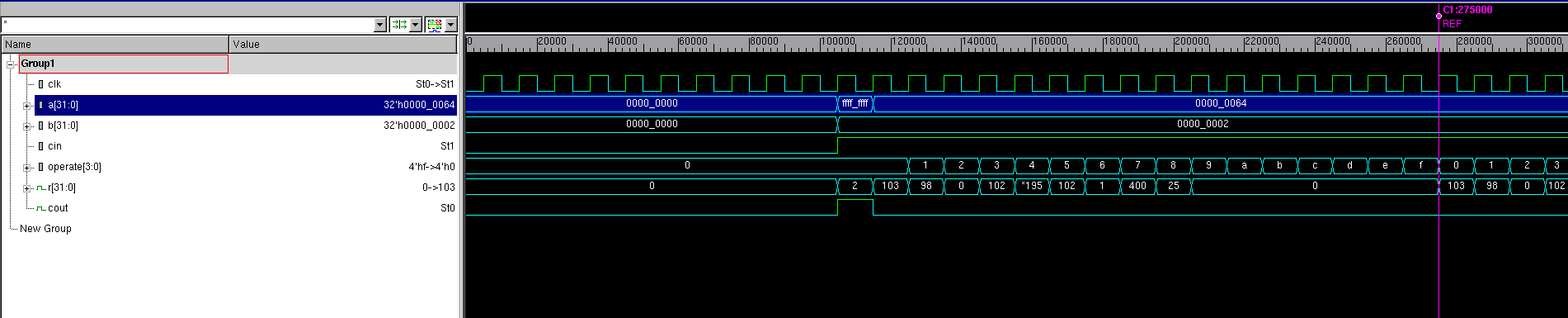展开全文verilog • 算术逻辑单元是中央处理器(CPU)执行单元，是所有中央处理器核心组成部分，由"And Gate"（与门） 和"Or Gate"（或门）构成算术逻辑单元，主要功能是进行二位元算术运算，如加减乘(不包括整数除法)。... 32位ALU设计 ​ 算术逻辑单元(arithmetic and logic unit) 是能实现多组算术运算和逻辑运算的组合逻辑电路，简称ALU。算术逻辑单元是中央处理器(CPU)的执行单元，是所有中央处理器的核心组成部分，由"And Gate"（与门） 和"Or Gate"（或门）构成的算术逻辑单元，主要功能是进行二位元的算术运算，如加减乘(不包括整数除法)。基本上，在所有现代CPU体系结构中，二进制都以补码的形式来表示。 设计思路 设计实现 仿真测试 文章目录32位ALU设计一、设计思路1. 算术运算2. 逻辑运算3.比较运算4.移位运算二、设计实现三、仿真测试四、模块设计1. CLA_adder32(1). 简单实现(2). 超前进位加法器(Carry-lookahead adder) 一、设计思路 1. 算术运算 addu、subu addu: 最高有效位向高位有进位，产生进位carryout subu: 当被减数小于减数时，最高有效位向高位有借位，产生借位carryout add、sub [x]补+[y]补=[x+y]补 [x-y]补=[x]补-[y]补=[x]补+[-y]补 [-y补]=~[y]补+1 add溢出：两个正数相加产生负数0111(7)+0110(6)=1101 ​ 两个负数相加产生正数1000(-8)+1001(-7)=0001 ​ 一个正数与一个负数相加不产生溢出 sub溢出：正数 - 正数（不产生溢出） ​ 正数 - 负数，结果为负数（上溢） ​ 负数 - 负数（不产生溢出） ​ 负数 - 正数，结果为正数（下溢） 2. 逻辑运算 ~：按位取反 &(and)：按位与操作 |(or)：按位或操作 ^(xor)：按位异或操作 ~(|)(nor)：按位或非操作 指令对标志位影响：指令执行后，CF和OF置0，ZF根据结果是否为0设置 3.比较运算 有符号数比较 slt 指令对标志位影响：in0小于in1置CF为1 in0为正数，in1为负数，out为0 in0为负数，in1为正数，out为1 in0和in1为负数，alu进行补码运算，1111（-1）、1110（-2），直接进行数值比较的结果与其代表的有符号数比较结果相同 in0和in1为正数，直接进行比较 通过分析，后两种情况可以合并 无符号数比较 sltu 可直接进行比较 指令对标志位影响：in0小于in1置OF为1 4.移位运算 逻辑左移shl (shift logical left)：sll，sllv 将数据向左移动，最低位用0补充 逻辑右移shr (shift logical right)：srl，srlv 将数据向右移动，最高位用0补充 算术右移sar (shift arithmetic right)：sra，srav 将各位依次右移指定位数，然后在左侧用原符号位补齐 指令对标志的影响：将最后移出的移位写入CF 总结来说，这六条移位操作指令可以分为两种情况：sllv、srav、srlv这3条指令的助记符最后有“v”，表示移位位数是通过寄存器的值确定的，sll、sra、srl这3条指令的助记符最后没有“v”，表示移位位数就是指令中6-10bit的sa的值。通过循环的方式，将移位后还在[31:0]部分的位置移到新的rd中，其他位置补上应补的0或1。 二、设计实现 module ALU32( op,in0,in1, carryout,overflow,zero,out ); input [31:0] in0,in1; input [10:0] op; output reg[31:0] out; output reg carryout,overflow,zero; always@(*) begin case(op) //add 11'b00000100000: begin out=in0+in1; overflow=((in0==in1)&&(~out==in0))?1:0; zero=(out==0)?1:0; carryout=0; end //addu 11'b00000100001: begin {carryout,out}=in0+in1; zero=(out==0)?1:0; overflow=0; end //sub 11'b00000100010: begin out=in0-in1; overflow=((in0==0&&in1==1&&out==1)|| (in0==1&&in1==0&&out==0))?1:0; zero=(in0==in1)?1:0; carryout=0; end //subu 11'b00000100011: begin {carryout,out}=in0-in1; zero=(out==0)?1:0; overflow=0; end //and 11'b00000100100: begin out=in0&in1; zero=(out==0)?1:0; carryout=0; overflow=0; end //or 11'b00000100101: begin out=in0|in1; zero=(out==0)?1:0; carryout=0; overflow=0; end //xor 11'b00000100110: begin out=in0^in1; zero=(out==0)?1:0; carryout=0; overflow=0; end //nor 11'b00000100111: begin out=~(in0|in1); zero=(out==0)?1:0; carryout=0; overflow=0; end //slt 11'b00000101010: begin if(in0==1&&in1==0) out=1; else if(in0==0&&in1==1) out=0; else out=(in0<in1)?1:0; overflow=out; zero=(out==0)?1:0; carryout=0; end //sltu 11'b00000101011: begin out=(in0<in1)?1:0; carryout=out; zero=(out==0)?1:0; overflow=0; end //shl 11'b00000000100: begin {carryout,out}=in0<<in1; overflow=0; zero=(out==0)?1:0; end //shr 11'b00000000110: begin out=in0>>in1; carryout=in0[in1-1]; overflow=0; zero=(out==0)?1:0; end //sar 11'b00000000111: begin out=($signed(in0))>>>in1;
carryout=in0[in1-1];
overflow=0;
zero=(out==0)?1:0;
end

endcase
end
endmodule

module ALU32_test(

);
reg [10:0] op;
reg [31:0] in0,in1;
wire [31:0] out;
wire carryout,overflow,zero;
ALU32 alu(op,in0,in1,carryout,overflow,zero,out);
initial
begin
op=11'b00000100000;
in0=32'hf2340000;
in1=32'h80000000;
#20  in0=32'h7fffffff;
in1=32'h70000001;
#20  in0=32'h7fffffff;
in1=32'hf0000001;
#20  in0=32'hffffffff;
in1=32'h00000001;
#20  op=11'b00000100001;
in0=32'hf2340000;
in1=32'h80000000;
#20  in0=32'h7fffffff;
in1=32'h70000001;
#20  in0=32'hffffffff;
in1=32'h00000001;
//sub
#20  op=11'b00000100010;
in0=32'h72340000;
in1=32'h60000000;
#20  in0=32'h7fffffff;
in1=32'hf0000001;
#20  in0=32'hf00fffff;
in1=32'h7ffffff1;
#20  in0=32'hffffffff;
in1=32'hffffffff;
#20  in0=32'hf0000000;
in1=32'h0fffffff;
//subu
#20  op=11'b00000100011;
in0=32'h72340000;
in1=32'h60000000;
#20  in0=32'h7fffffff;
in1=32'hf0000001;
#20  in0=32'hffffffff;
in1=32'hffffffff;
#20  in0=32'hf0000000;
in1=32'h0fffffff;
//and
#20  op=11'b00000100100;
in0=32'h72340000;
in1=32'h60000000;
#20  in0=32'h7fffffff;
in1=32'h00000000;
//or
#20  op=11'b00000100101;
in0=32'h00000000;
in1=32'h00000000;
#20  in0=32'h7fffffff;
in1=32'hf0000001;
//xor
#20  op=11'b00000100110;
in0=32'ha0000000;
in1=32'h50000000;
#20  in0=32'h7fffffff;
in1=32'hf0000001;
//nor
#20  op=11'b00000100111;
in0=32'h123451ff;
in1=32'h60000000;
#20  in0=32'h7fffffff;
in1=32'hf0000001;
//slt
#20  op=11'b00000101010;
in0=32'h72340000;
in1=32'hf0000000;
#20  in0=32'h7000000f;
in1=32'h7f000001;
#20  in0=32'hf0001231;
in1=32'h7ac34545;
//sltu
#20  op=11'b00000101011;
in0=32'h72340000;
in1=32'hf0000000;
#20  in0=32'h7000000f;
in1=32'h7f000001;
#20  in0=32'hf0001231;
in1=32'h7ac34545;
//shl
#20  op=11'b00000000100;
in0=32'hffffffff;
in1=32'd5;
//shr
#20  op=11'b00000000110;
in0=32'hffffffff;
in1=32'd5;
//sar
#20  op=11'b00000000111;
in0=32'hffffffff;
in1=32'd3;
#20  in0=32'h0fffffff;
in1=32'd5;
end

endmodule

三、仿真测试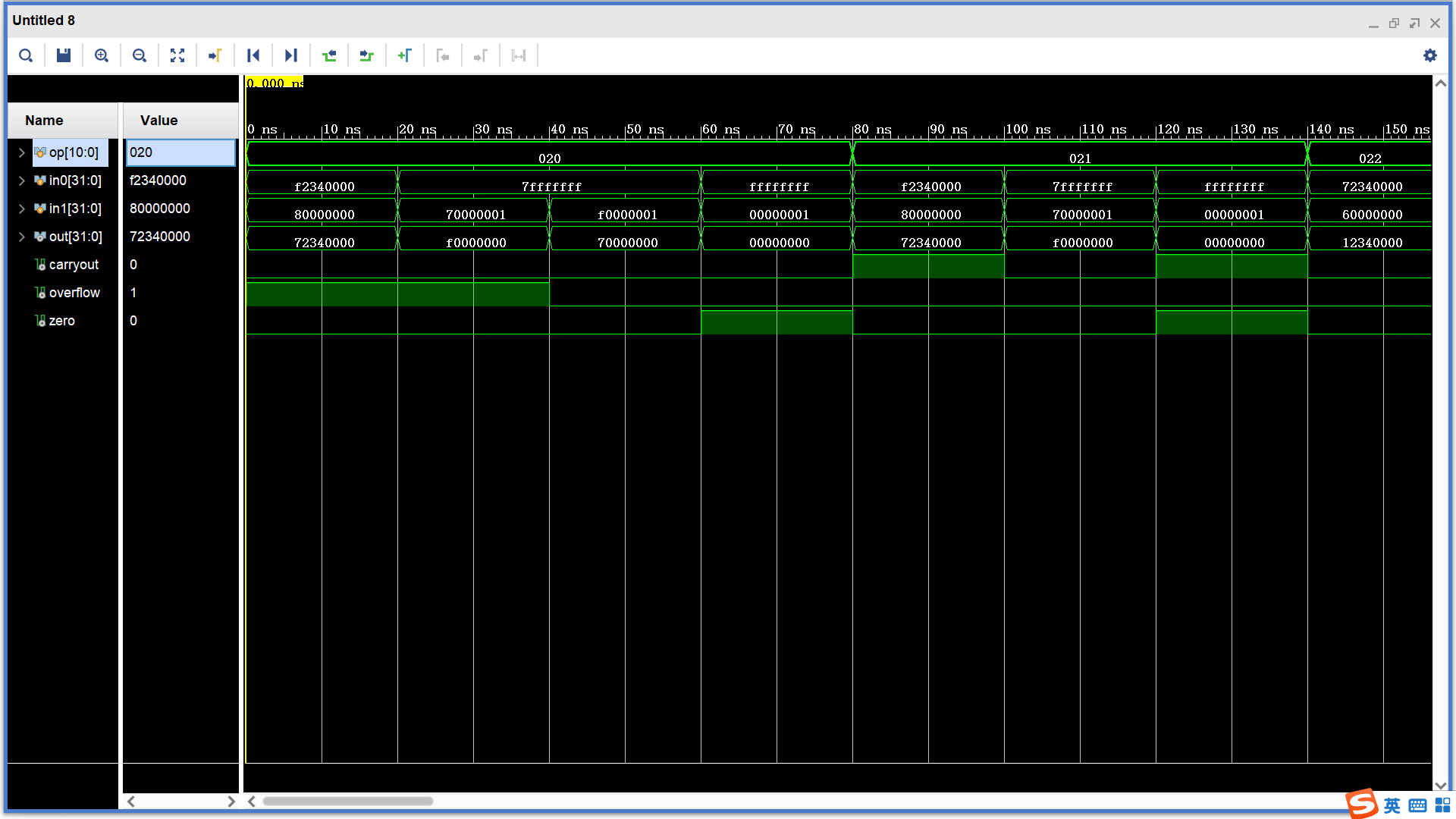sub、subu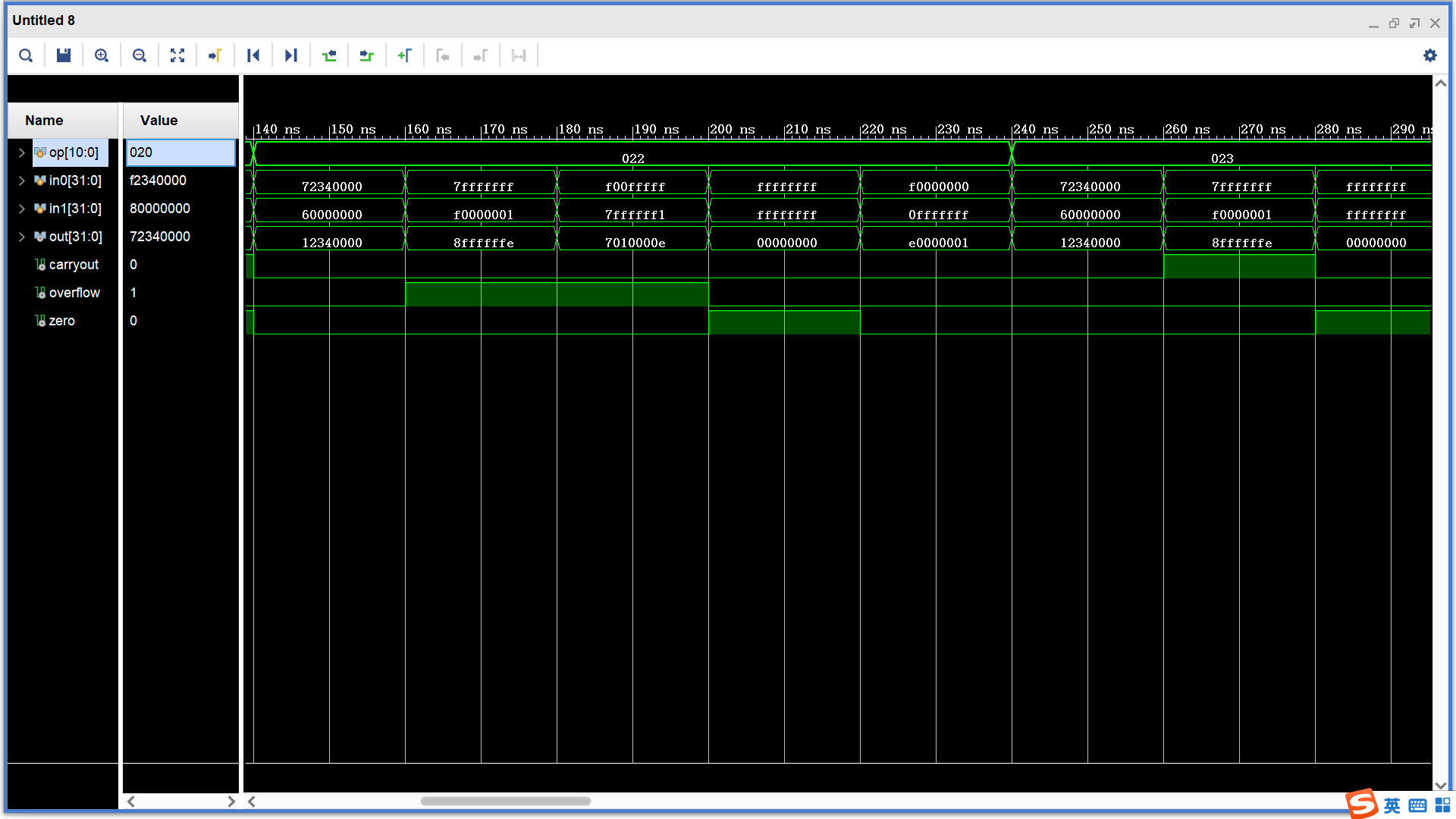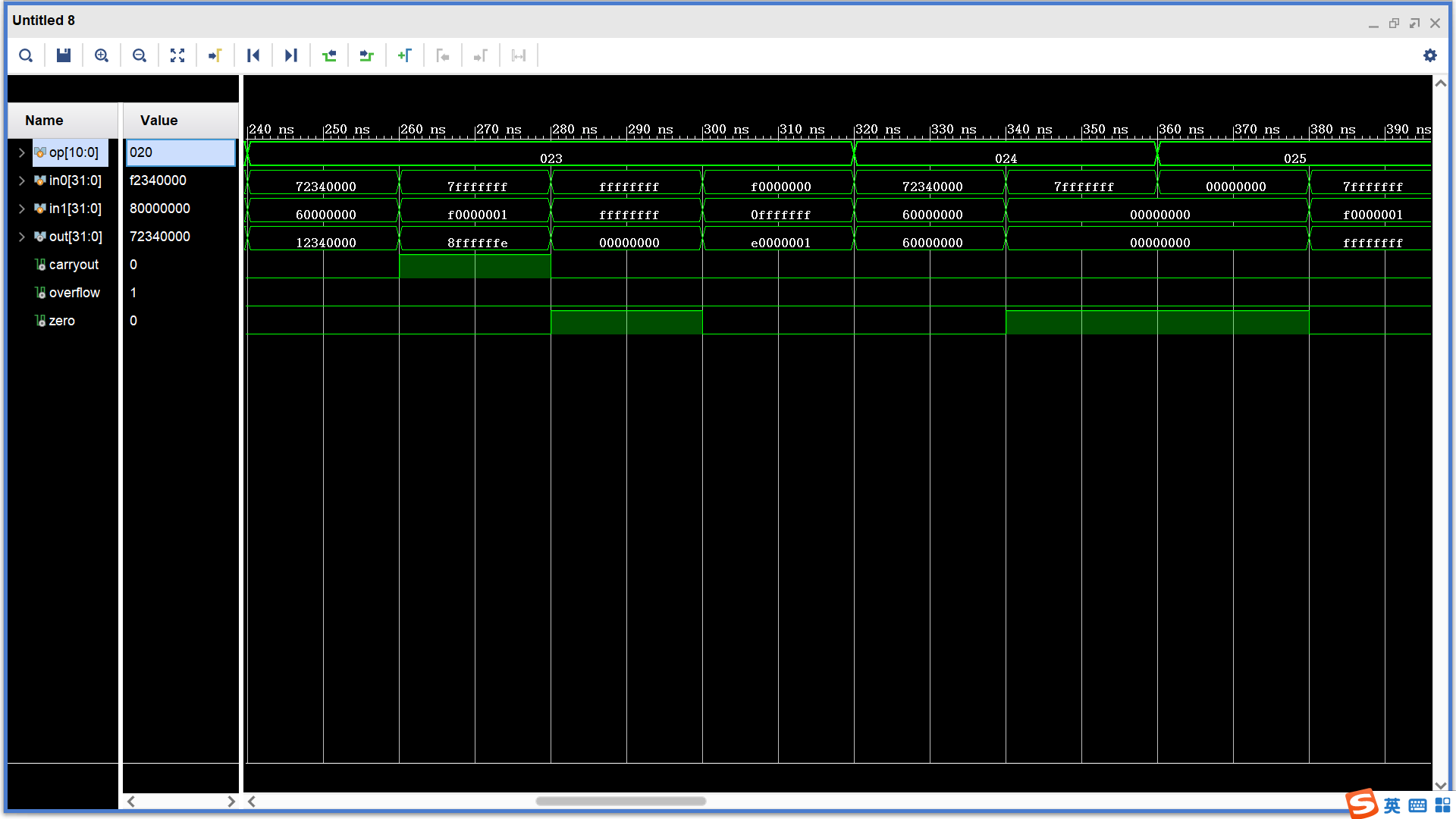and、or、xor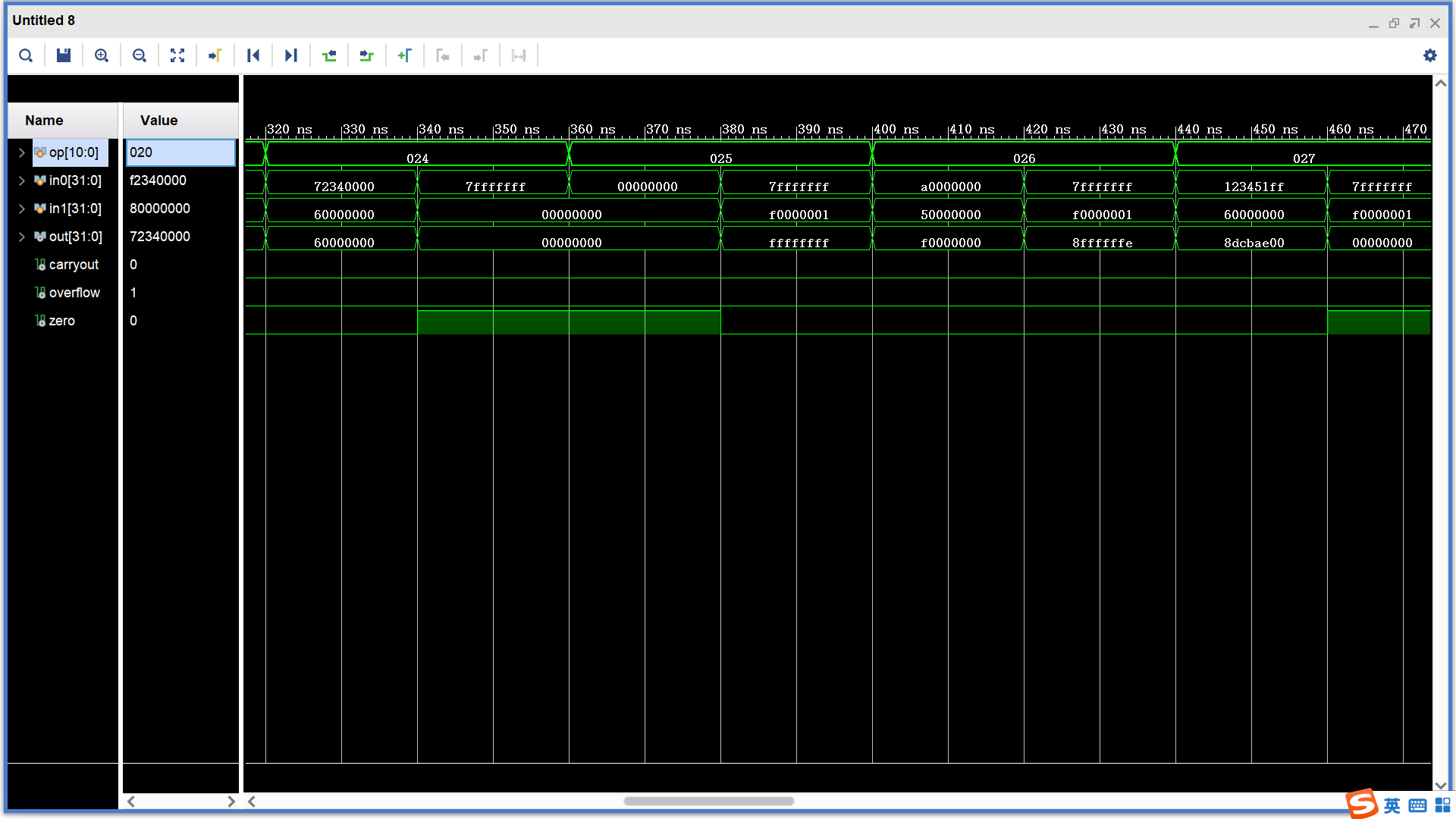nor、slt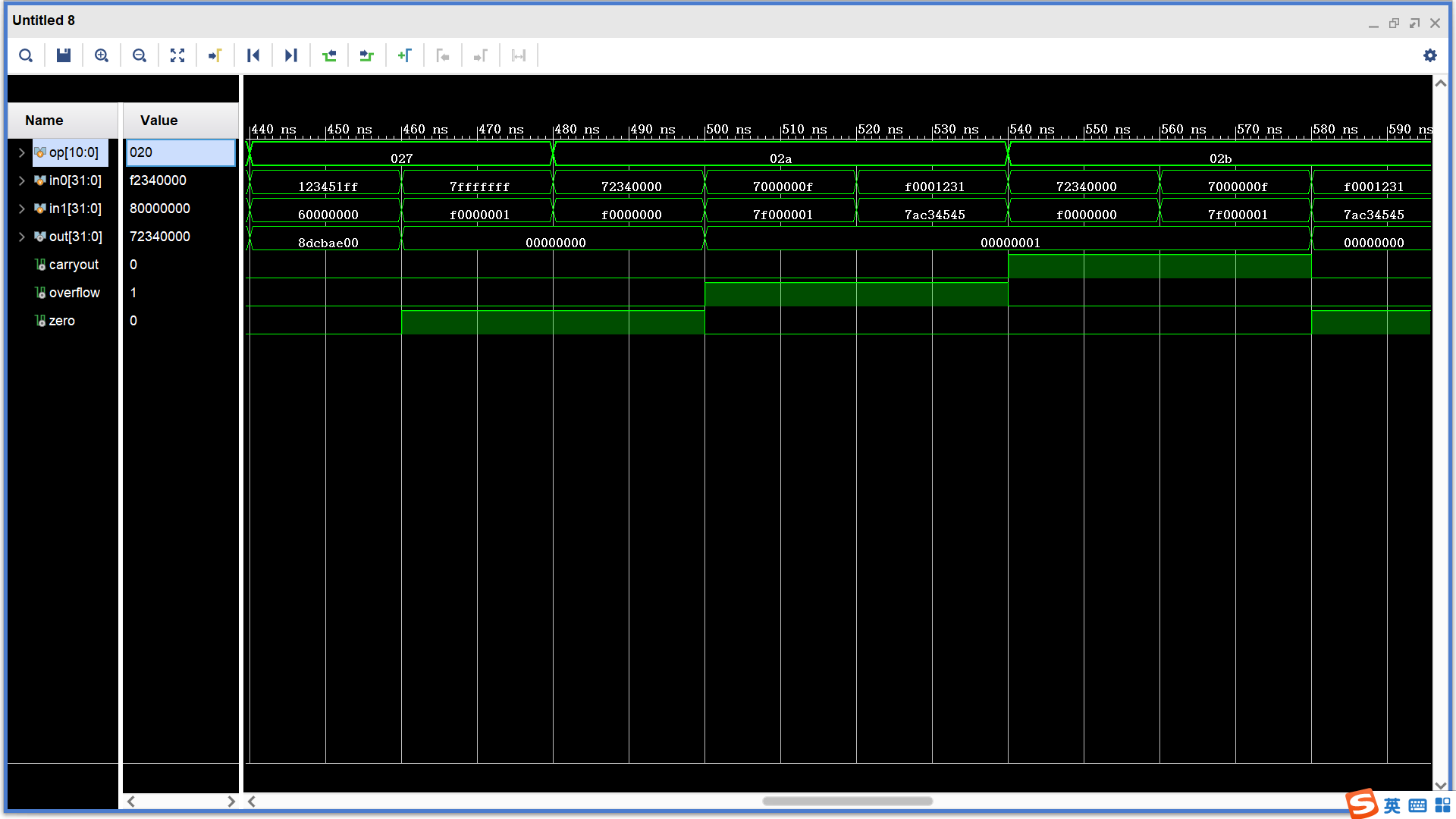sltu、shl、shr、sar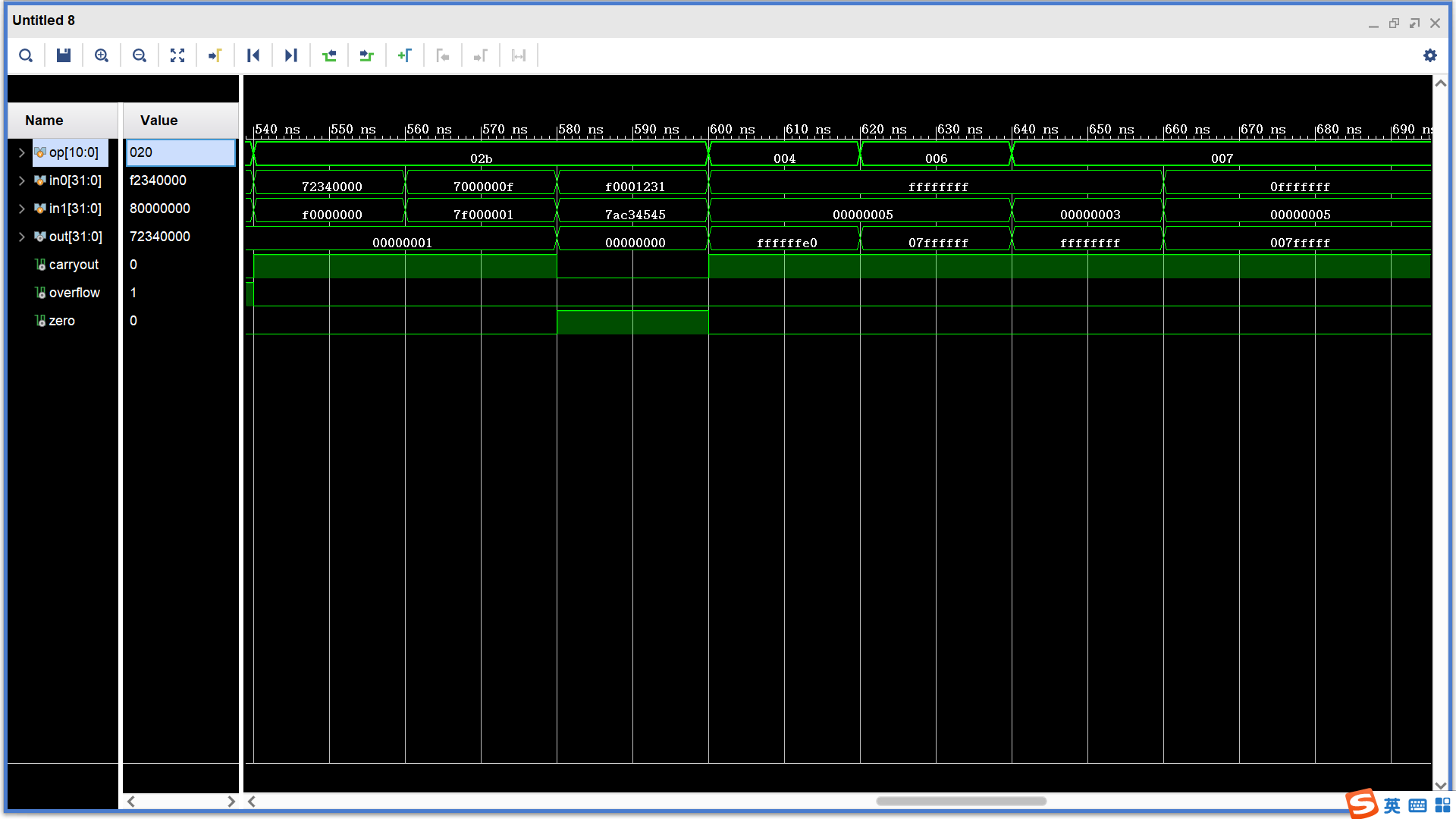四、模块设计
(1). 简单实现
  module full_adder(
cin,x,y,cout,s
);
input [31:0] x,y;
input cin;
output cout;
output [31:0] s;
assign {cout,s}=cin+x+y;
endmodule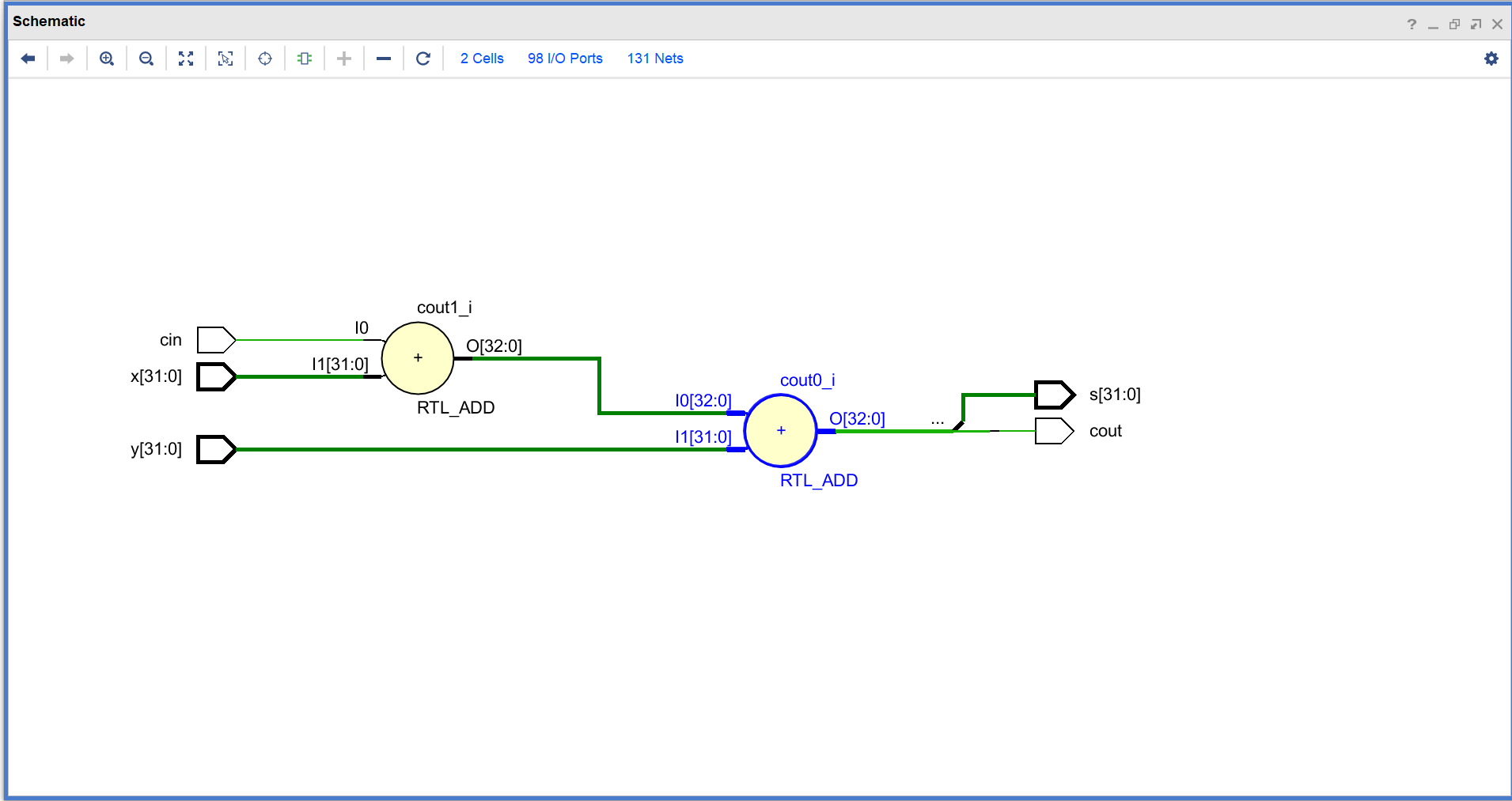这里在综合后使用了两个32位全加器，实际门延迟比32位全加器高。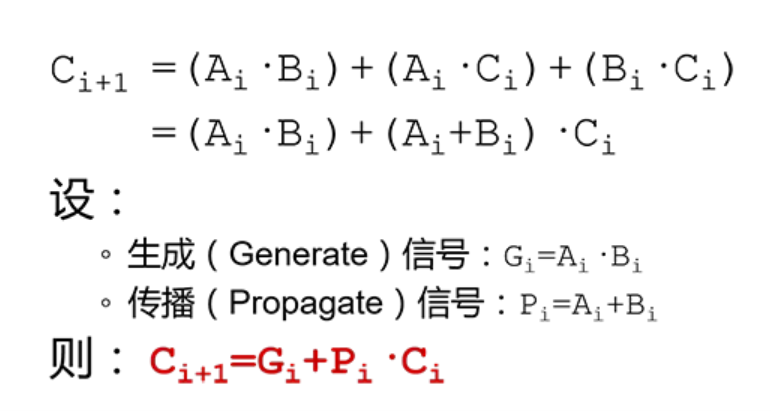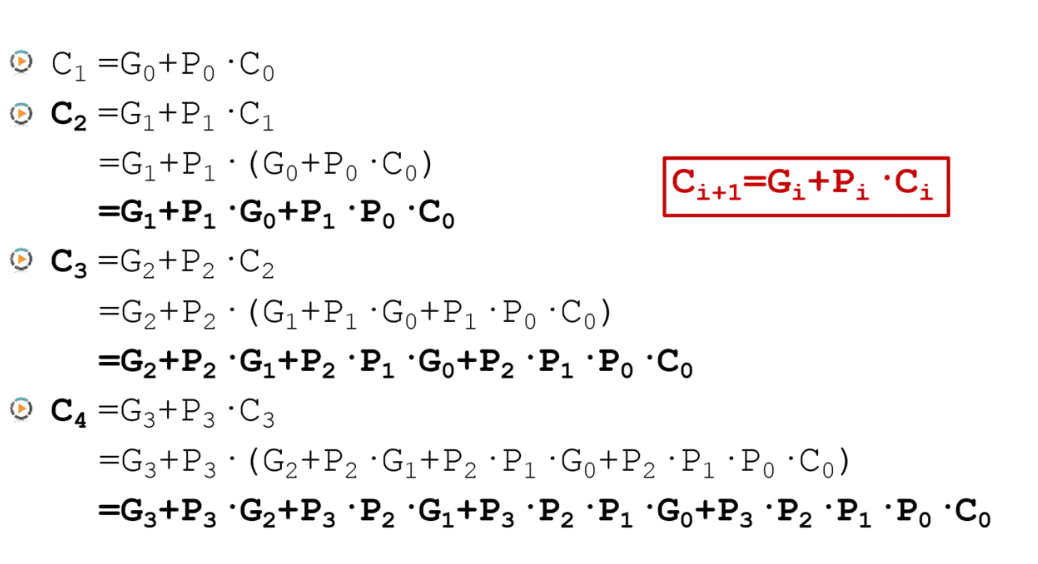加法器的优化——超前进位加法器
由上式可以看出直接实现32位超前进位加法器会非常复杂，所以首先实现8位超前进位加法器，并通过4个8位超前进位加法器组成32位超前进位加法器。
module CLA_adder8(
cin,a,b,
cout,s
);
input [7:0] a,b;
input cin;
output [7:0] s;
output cout;
wire [7:0] G,P;
wire [7:0] C;

assign G=a&b;
assign P=a|b;
assign C=cin;
assign s=a^b^C;

assign G=a&b;
assign P=a|b;
assign C=G|(P&cin);
assign s=a^b^C;

assign G=a&b;
assign P=a|b;
assign C=G | (P&G) | (P&P&cin);
assign s=a^b^C;

assign G=a&b;
assign P=a|b;
assign C=G | (P&G) | (P&P&G) | (P&P&P&cin);
assign s=a^b^C;

assign G=a&b;
assign P=a|b;
assign C=G | (P&G) | (P&P&G) | (P&P&P&G) |    						(P&P&P&P&cin);
assign s=a^b^C;

assign G=a&b;
assign P=a|b;
assign C=G | (P&G) | (P&P&G) | (P&P&P&G) | 						(P&P&P&P&G) |
(P&P&P&P&P&cin);
assign s=a^b^C;

assign G=a&b;
assign P=a|b;
assign C=G | (P&G) | (P&P&G) | (P&P&P&G) | 						(P&P&P&P&G) |
(P&P&P&P&P&G) | (P&P&P&P&P&P&cin);
assign s=a^b^C;

assign G=a&b;
assign P=a|b;
assign C=G | (P&G) | (P&P&G) | (P&P&P&G) | 						(P&P&P&P&G) |(P&P&P&P&P&G) | 							(P&P&P&P&P&P&G) |
(P&P&P&P&P&P&P&cin);
assign s=a^b^C;

assign cout=G | (P&G) | (P&P&G) | (P&P&P&G) | 						(P&P&P&P&G) |(P&P&P&P&P&G) | 							(P&P&P&P&P&P&G) |
(P&P&P&P&P&P&P&G) |
(P&P&P&P&P&P&P&P&cin);
endmodule

仿真测试：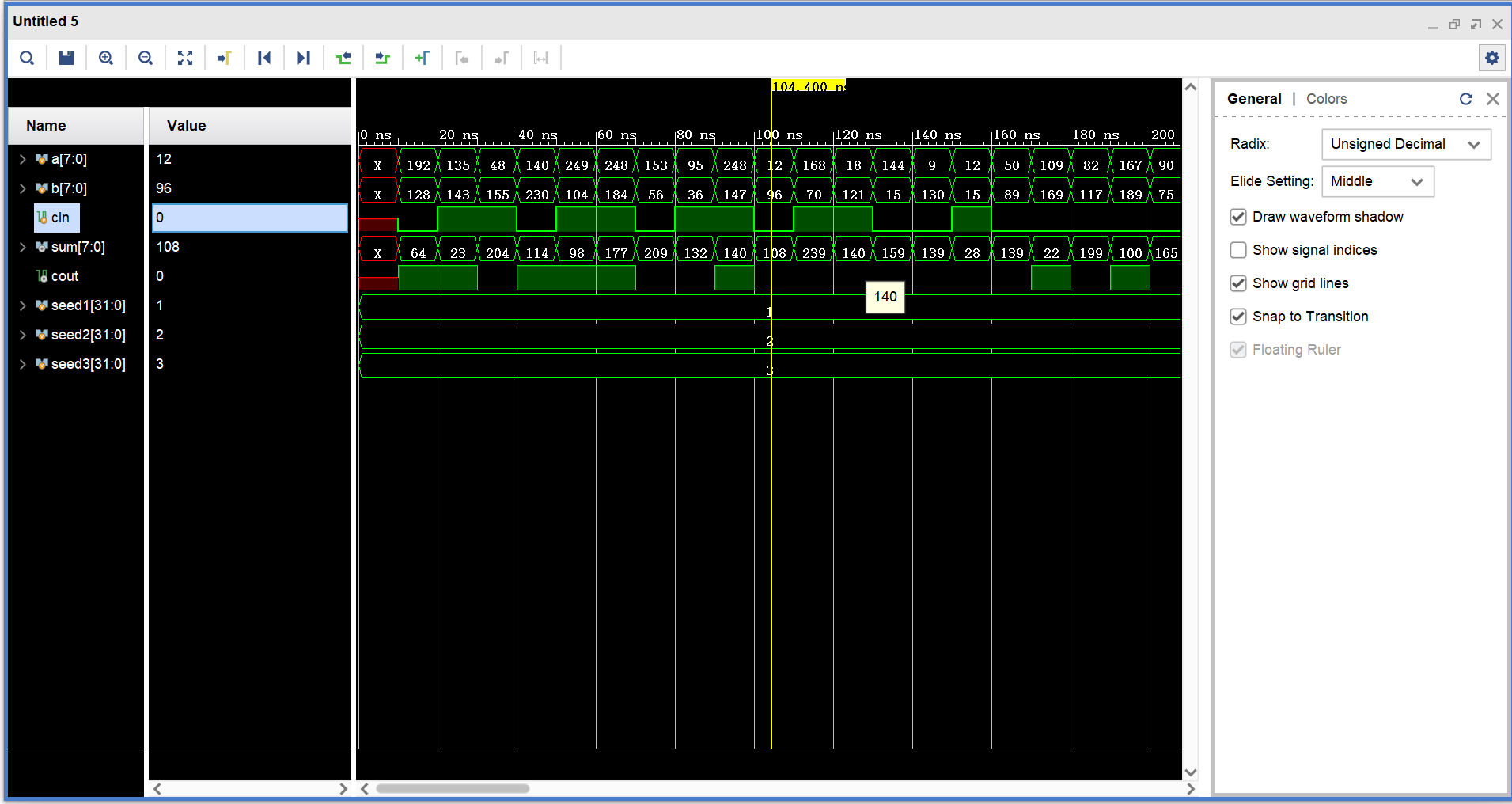门延迟分析：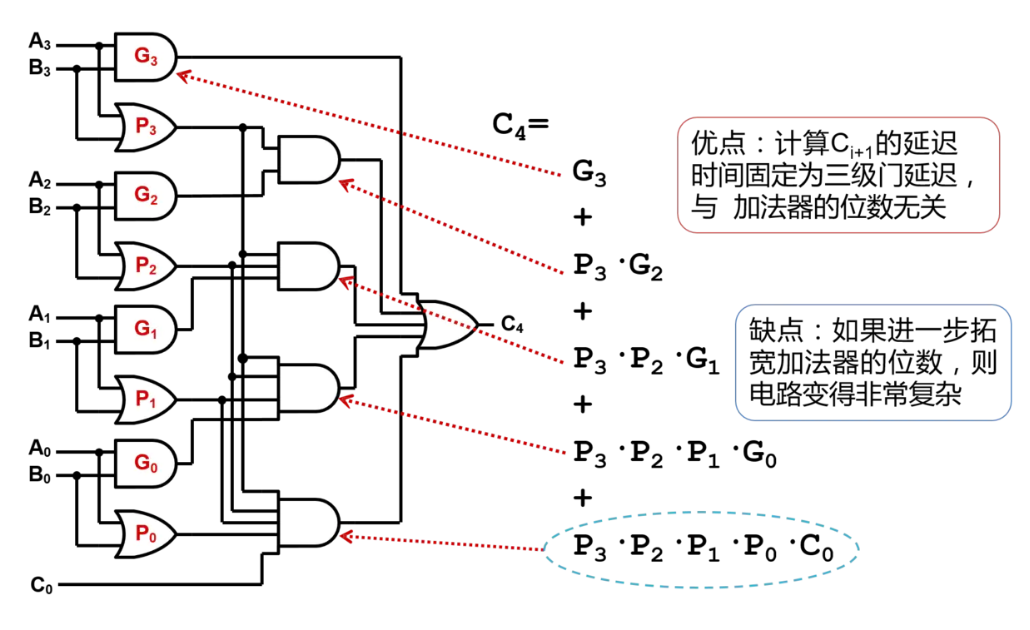4级8位超前进位加法器构成的32位超前进位加法器门延迟：3*4+1=13
module CLA_adder32(
cin,x,y,
cout,s
);
input [31:0] x,y;
input cin;
output [31:0] s;
output cout;
wire cout1,cout2,cout3;
endmodule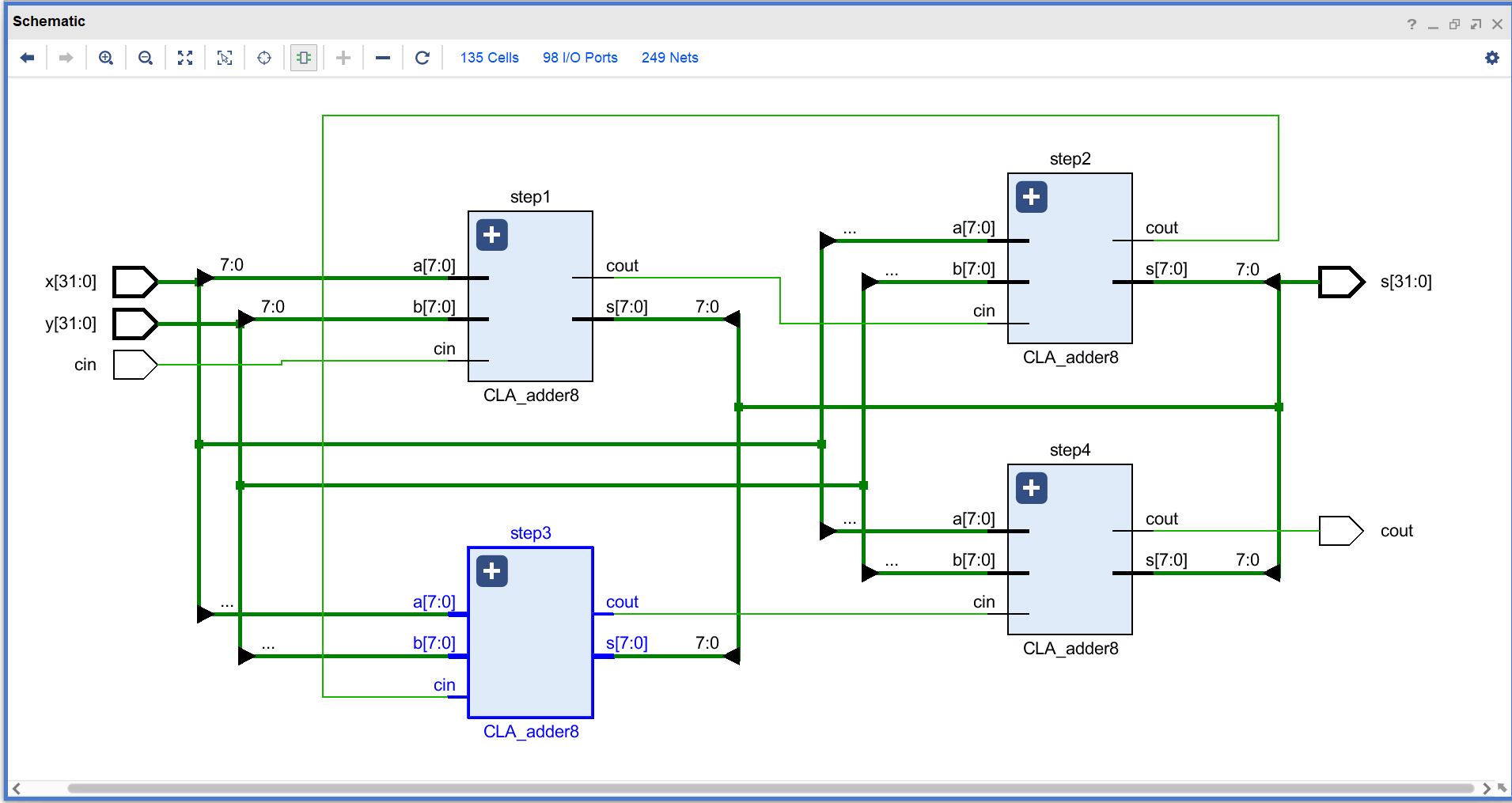仿真测试：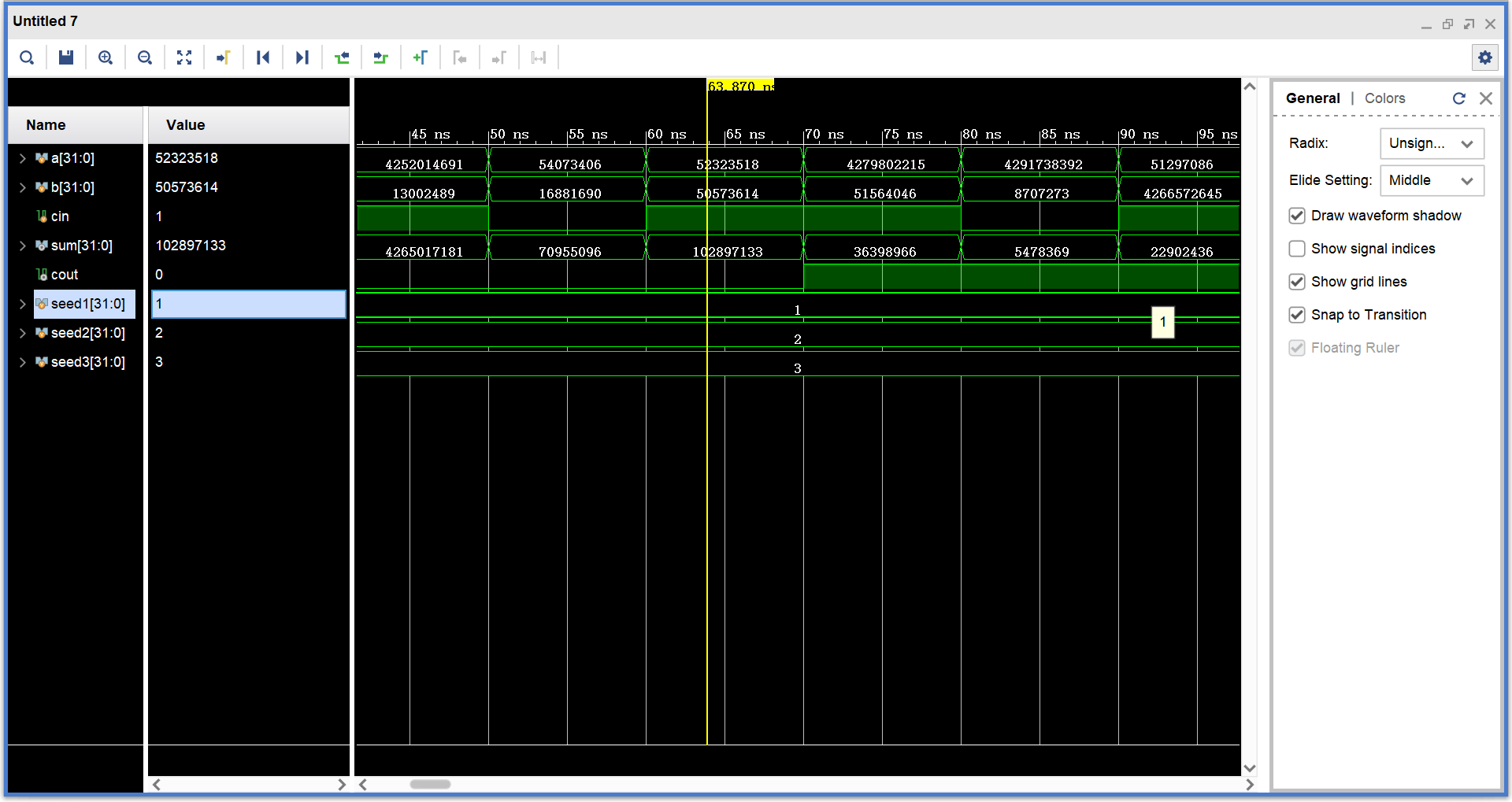展开全文verilog
• 算术逻辑单元（Arithmetic&logical Unit）是中央处理器(CPU)执行单元，是所有中央处理器核心组成部分，由"And Gate"（与门） 和"Or Gate"（或门）构成算术逻辑单元，主要功能是进行二位元算术运算，如加减乘...
• 内部有一个8位的，同时知道了CPU内部包含了运算器，控制器及若干寄存器。51单片机CPU的内部结构及工作原理。... 图片11、运算器（ALU的主要功能 A）算术和逻辑运算，可对半字节（一个字节是8位，半个字节就是4位）
• CPU相关知识 ...主要由两部分构成，控制单元 和 算数逻辑单元（ALU）。 控制单元：从内存提取指令并解码执行。 算数逻辑单元：处理算数和逻辑运算 从功能来看，CPU内部结构为： 寄存器：可以用来
CPU相关CPU是什么CPU做什么CPU的内部结构CPU是寄存器的集合体计算机语言寄存器的分类&汇编语言程序计数器标志寄存器函数调用机制通过地址和索引实现数组CPU指令执行过程
CPU是什么
Central Processing Unit，小型计算机芯片，嵌在主板上。
CPU做什么
CPU的核心是从程序或应用程序获取指令并执行运算，分为：提取、解码和执行三个关键阶段。
CPU从系统的RAM中提取指令 然后解码该指令的实际内容，然后再由CPU相关部分执行该指令。
CPU的内部结构
主要由两部分构成，控制单元  和  算数逻辑单元（ALU）。

控制单元：从内存中提取指令并解码执行。
算数逻辑单元：处理算数和逻辑运算

从功能来看，CPU内部结构为：

寄存器：可以用来暂存指令、数据和地址。可以将其看作是内存的一种。
控制器：负责把内存上的指令、数据读入寄存器，并根据指令的结果控制计算机。
运算器：负责运算从内存中读入寄存器的数据。
时钟：负责发出CPU开始计时的时钟信号。

CPU是寄存器的集合体
计算机语言
低级语言：汇编语言和机器语言
高级语言：如C、JAVA等
寄存器的分类&汇编语言
寄存器分类：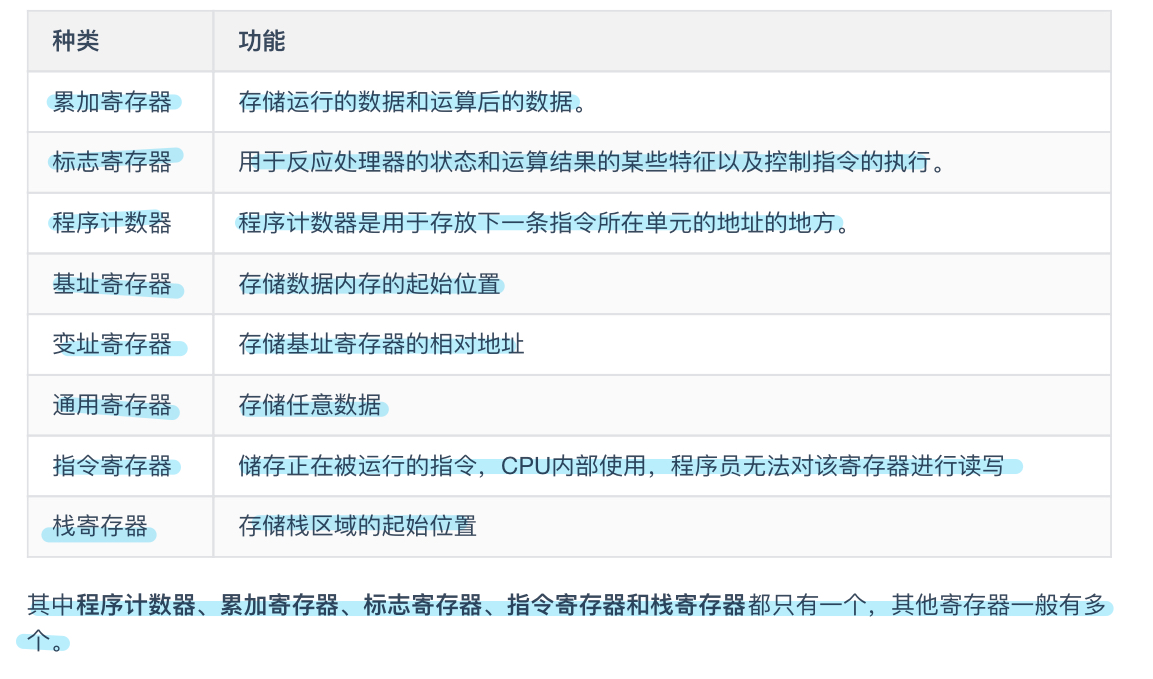程序计数器
Program Counter 用来存储下一条指令所在的单元地址。
程序执行时，PC的初值为程序第一条指令的地址，在顺序执行程序时，控制器首先按照程序计数器指出的指令地址从内存中取出一条指令，然后分析和执行该指令，同时将PC的值加1指向下一条要执行的指令。
程序计数器控制着程序的流程。
标志寄存器
保存累加寄存器的运算结果，也负责溢出和奇偶校验。
运算结果有正、负、零三种，第一个字位、第二个字位、第三个字位各自的结果为1时，分别代表正、负和零。
程序比较指令，实际上是CPU内部做减法运算。
函数调用机制
函数的调用和返回很重要的两个指令是call和return指令。在将函数的入口地址设定到程序计数器之前，call指令会把调用函数后要执行的指令地址存储在名为栈的主存内。函数处理完毕后，再通过函数的出口来执行return指令。
通过地址和索引实现数组
实际地址 = 基址寄存器的值 +  变址寄存器的值
（固定：100000000） （变化）
CPU指令执行过程展开全文操作系统
• MAC单元XC166中是以一个算法处理单元出现，类似于CPU中的算术逻辑（ALU）单元。这种结构优点是可以保持XC166与C166兼容性。 MAC单元有自己寻址模式和指令集，MAC指令集是专为开发DSP程序而设计。独立...
• MAC单元XC166中是以一个算法处理单元出现，类似于CPU中的算术逻辑（ALU）单元。这种结构优点是可以保持XC166与C166兼容性。  MAC单元有自己寻址模式和指令集，MAC指令集是专为开发DSP程序而设计。...
• (1) 选定CPU中所使用产要器件； (2) 根据指令系统、选用器件和设计指标，设计指令流数据通路； (3) 根据指令系统、选用器件和设计指标，设计数据流数据通路。 计算机工作过程，实质上是不同数据流...
• Hack平台的大部分行为都发生在CPU中，因此主要任务就是构建CPU，其他的主要是正确连接。 CPU CPU的实现目标是建立逻辑门结构，使其能执行指定的HACK指令和读取下一条要执行的指令。 CPU包括： ALU，执行HACK指令 一...
• 计算机硬件组成（运维关注的重要部分）：CPU：中央处理器，是一个计算机的运算核心和控制核心，他的主要功能是解释计算机指令以及处理计算机软件的数据。 中央处理器主要包括：运算器 高速缓冲存储器(ALU) （cache...
• 中央处理器CPU是单片微型计算机指挥、执行中心，由它读人用户程序，并逐条执行指令，它是由8位算术／逻辑运算部件（简称ALu）、定时／控制部件，若干寄存器A、B、B5w、5P以及16位程序计数器（Pc）和数据指针...
• 计算机是一个统称，实现计算部件是CPU，在CPU内部有一个ALU 维基介绍 　算术逻辑单元（英语：Arithmetic Logic Unit, ALU）是中央处理器执行单元，是所有中央处理器核心组成部分，由与门和或门构成算数...
• 8086 由执行单元EU 和 总线结构单元BIU 两大部分构成。 执行单元EU 主要功能是：执行指令、分析指令、暂存中间运算...总线接口单元 BIU 主要功能是：负责CPU与存储器、I/O接口之间信息传送。它由段寄存器、指令指PSW寄存器
• 注： 下列所示代码与题目可能有些出入，xor，sub等功能在作者之前文章均已经实现，可见之前文章 R-I CPU设计实验。本代码主要侧重 lw_inc 功能的实现，主要通过修改REG模块，其中加入一个控制信号以实现rs加1...
• 的功能主要是解释计算机指令以及处理计算机软件中的数据。中央处理器主要由三核心部件组成，运算器、控制器和总线(BUS)，运算器又主要由算术逻辑单元(ALU)和寄存器(RS)组成。 　CPU Cache(缓存区)即高速缓冲...
• 算术逻辑单元 (Arithmetic Logic Unit, ALU)是中央处理器(CPU)执行单元，是所有中央处理器核心组成部分，由"与门" 和"或门"构成算术逻辑单元，主要功能是进行二进制算术运算，如加减乘(不包括整数除法)。...
• 特点是速度快，随时修改，随时读取，掉电后所有数据丢失SRAM ：静态随机访问存储 cpu中cache(缓存)就是这种类型，51单片机内存是这种类型2kB，4kB，体积大，价格高，没有作为大容量存储设备，它存取速度比...
• 试题1 CPU主要由运算单元（ALU）、控制单元（CU）、寄存器和时序电路组成。指令执行过程存放指令、操作数和中间结果的功能是由 （1） 实现。 （1） A．运算单元 B．控制单元 C．寄存器 D．时序电路
• CPU应具备哪些主要功能？ 答：1.CPU在内部结构上由以下几部分组成： ① 算术逻辑部件(ALU)； ② 累加器和通用寄存器组； ③ 程序计数器(指令指针)、指令寄存器和译码器； ④ 时序和控制部件。 2.CPU应具备以下主要...
• 一、8086微处理器内部结构 ...总线接口部件(BIU)主要由地址加法器、专用寄存器组、指令队列和总线控制电路4个部件组成，其主要功能是形成访问存储器物理地址、访问存储器并取指令暂存到指令队列等待执行，访问存储
•  主存：计算机存放正在运行的程序和数据的存储器，为计算机的主要工作存储器，可随机存取；由存储体、各种逻辑部件及控制电路组成。  存储单元：可存放一个机器字并具有特定存储地址的存储单位。  存储元件...
• Logisim中的主要组成部分之一就在于设计并以图示来显示CPU。当然Logisim还有其他多种组合分析模型来对你进行帮助，如转换电路，表达式，布尔型和真值表等等。同时还可以重新利用小规模的电路来作为大型电路的一...
• 8． 计算机使用总线结构的主要优点是便于实现积木化，同时______。c A 减少了信息传输量 B 提高了信息传输的速度 C 减少了信息传输线的条数 D 加重了CPU的工作量 9． 带有处理器的设备一般称为______设备。a A 智能...
• 3． 运算器的主要功能是进行______。 A.逻辑运算 B.算术运算 C.逻辑运算与算术运算 D.初等函数的运算 4． 某计算机字长16位，它的存贮容量是64K，若按字编址，那么它的寻址范围是______。 A.64K B.32K C.64KB D.32KB...计算机组成原理